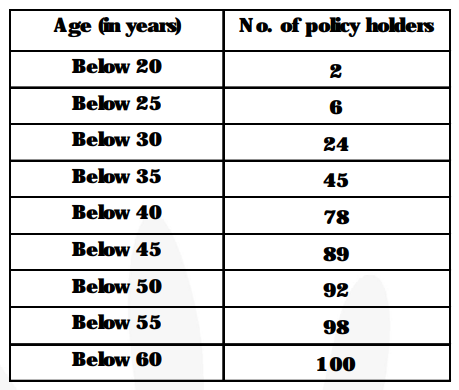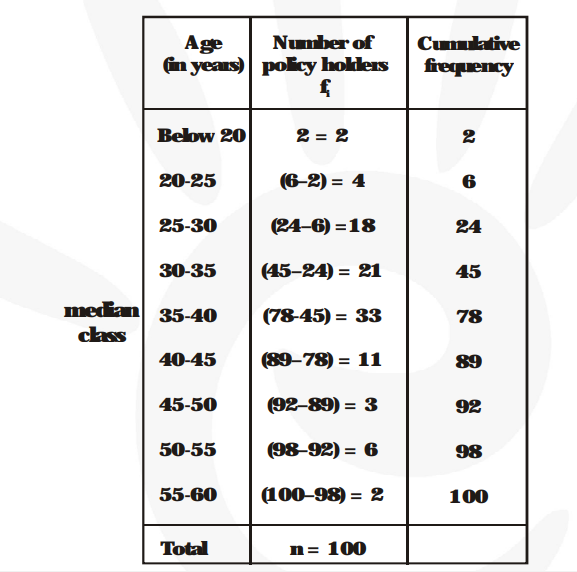# A life insurance agent found the following data for distribution of ages of 100 policy holders.

Question:

A life insurance agent found the following data for distribution of ages of 100 policy holders. Calculate the median age, if policies are only given to persons having age 18 years onwards but less than 60 year.Solution:Here, $\ell=35, \mathrm{n}=100, \mathrm{f}=33, \mathrm{cf}=45, \mathrm{~h}=5$

Median $=\ell+\left\{\frac{\frac{\mathbf{n}}{\mathbf{2}}-\mathbf{c f}}{\mathbf{f}}\right\} \times \mathrm{h}$

$=35+\left\{\frac{\mathbf{5 0}-\mathbf{4 5}}{\mathbf{3 3}}\right\} \times 5$

$=35+\frac{\mathbf{2 5}}{\mathbf{3 3}}$

= 35 + 0.76

= 35.76 years.